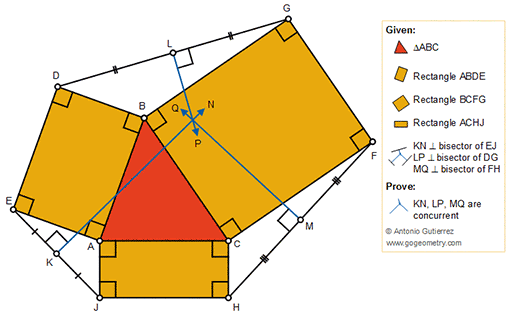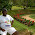Tuesday, November 13, 2018

Geometry Problem 1400: Triangle with three rectangles on the sides, Perpendicular Bisectors, Concurrency

Geometry Problem. Post your solution in the comment box below.
Level: Mathematics Education, High School, Honors Geometry, College.

Details: Click on the figure below.1.Let KN & LP meet at O

Let OB = u, OA = v & OC = w
Let OE = OJ = a, OD = OJ = b, OH = c & OF = d

Using Apollonius for Tr. OBE & ODJ,

2.OP^2 + 2.BP^2 =
a^2 + u^2 = b^2 + v^2 ...(1)

Similarly

b^2 + w^2 = d^2 + u^2 ...(2) and
c^2 + v^2 = a^2 + w^2 ....(3)

Adding (1), (2), (3) gives c = d

So MQ must pass thro O

Sumith Peiris
Moratuwa
Sri Lanka

1.Very nice solution Sumith

2.3.